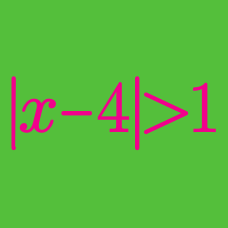Algebra

# Absolute Value Inequalities: Level 3 Challenges

$\left | 1 - \frac{|x|}{1 + |x|} \right| \geq \frac{1}{2}$

The solution set to this inequality is $$a \le x \le b.$$ What is $$b-a?$$

How many integers $$x$$ satisfy the inequality $$|x-2000|+|x| \leq 9999$$?

How many ordered pairs of integers $$(x,y)$$ are there that satisfy $$|x| + |y| \leq 10$$?

Consider all monic polynomials $$f(x) = x^2 + bx + c$$, where $$b$$ and $$c$$ are real numbers. What is the minimum value of $$N$$, where

$N = \max_{x \in [-10,10]} \vert f(x) \vert?$

Details and assumptions

The last equation states: "The maximum value of the absolute value of $$f(x)$$ , as $$x$$ ranges from $$-10$$ to $$10$$ inclusive".

$\large 2=|x-2|+|x-4|$

Find the solution set of the above equation.

×

Problem Loading...

Note Loading...

Set Loading...